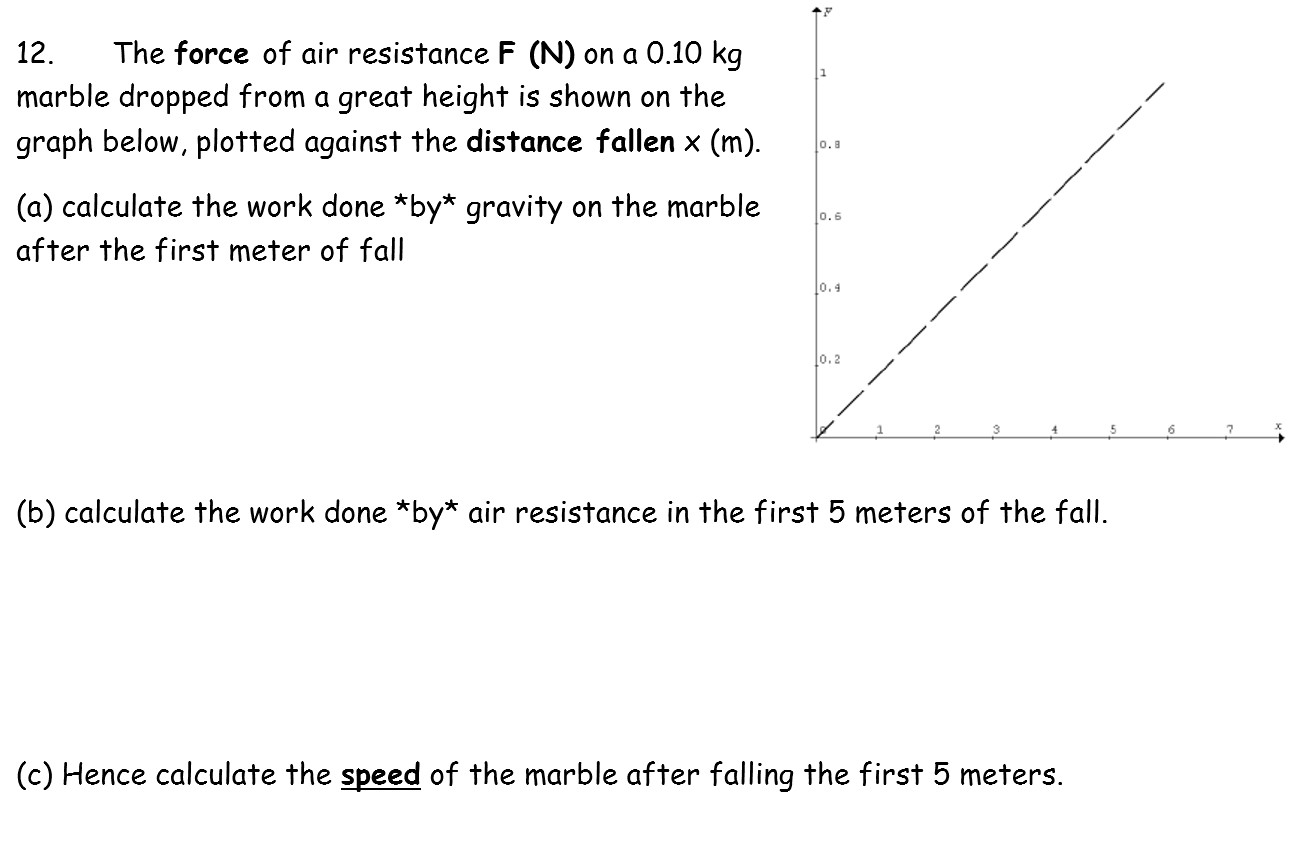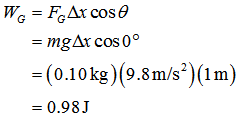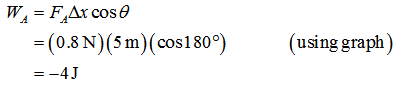# The force of air resistance F (N) on a 0.10 kgmarble dropped from a great height is shown on thegraph below, plotted against the distance fallen x (m).12.o.8(a) calculate the work done *by* gravity on the marble0.6after the first meter of fall0.4l0.2(b) calculate the work done *by* air resistance in the first 5 meters of the fall.(c) Hence calculate the speed of the marble after falling the first 5 meters.

Question
76 viewshelp_outlineImage TranscriptioncloseThe force of air resistance F (N) on a 0.10 kg marble dropped from a great height is shown on the graph below, plotted against the distance fallen x (m). 12. o.8 (a) calculate the work done *by* gravity on the marble 0.6 after the first meter of fall 0.4 l0.2 (b) calculate the work done *by* air resistance in the first 5 meters of the fall. (c) Hence calculate the speed of the marble after falling the first 5 meters. fullscreen
check_circle

Step 1

(a) Work done by gravity is,Step 2

(b) Work done by air resistance is,...

### Want to see the full answer?

See Solution

#### Want to see this answer and more?

Solutions are written by subject experts who are available 24/7. Questions are typically answered within 1 hour.*

See Solution
*Response times may vary by subject and question.
Tagged in